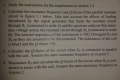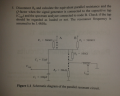# How to calculate resonance frequency and Q factor in my resonant circuit

#### Ali Alkhudri

Joined Nov 3, 2015
19
Hi
I have some questions about resonant circuits , I started this course in last week .. In the piture below I want to calculate resonance frequency
and Q factor , How to do that?For question 1) I red the book
For question 2) What I know that W = 1/sqrt(C*L) , but according to the question I have to take into account R1 and R2 , How to do that and What formula I have to use??
Q factor is Q=Rp/W*L , but this does not fit , it feels that I have to use another formula?
For question 3) I do not understand it
For question 4) Q=W*L/Rs , it it correct?
For question 5) How to think here? the question is not clear

thanks

#### Ali Ali 1

Joined Nov 8, 2015
2
We did calculate it .. I will upload the solutions later#### JoeJester

Joined Apr 26, 2005
4,390

#### MrAl

Joined Jun 17, 2014
10,621
Hi,

I dont know if this will help here but, to find the resonant frequency for a general network you can find the critical point(s) of the transfer function. Once you have the resonant frequency you can then find the upper and/or lower 3db cutoff frequencies and that will allow you to calculate the circuit Q.
This method is not that easy to do sometimes when the order gets high, but it is a general method which should work with any network as long as you can simplify and solve the resulting equations. If you have been taught a simpler method you should try to apply that first.